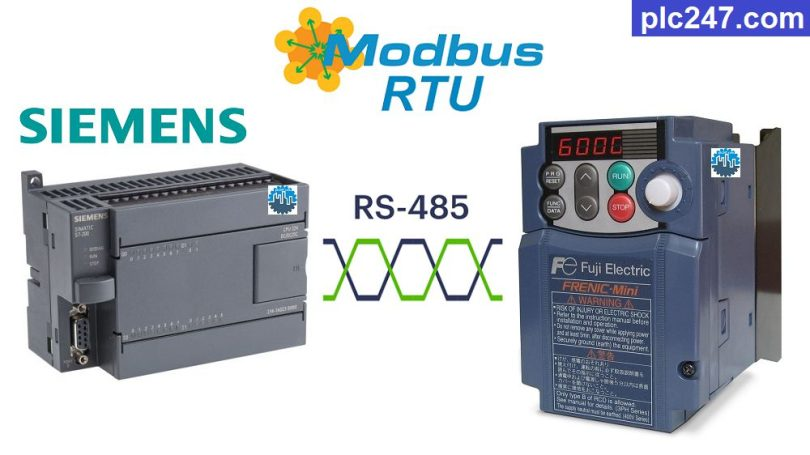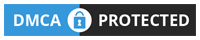# Siemens S7-200 “Modbus RTU” Fuji Frenic VFDWritten by

Hello everyone, continuing the series using Siemens S7-200 PLC to control different types of inverters through Modbus-RTU communication protocol, today plc247.com continues to guide you to control Fuji Frenic inverters.

The inverter model used is Fuji Frenic Mini (similar ECO, Multi models) I will guide you to control it in the simplest and easiest way.

#### Siemens S7-200 & Fuji Frenic “Wiring Diagram”

RS485 communication connection diagram between PLC Siemens S7-200 and Fuji Frenic Mini inverter.

#### Fuji Frenic Modbus Parameters Setting

Below are the Modbus communication parameters that need to be installed for the Fuji Frenic inverter:

• H30: 3 (VFD Control via RS485 Port)
• Y02: 0
• Y03: 2
• Y04: 2 (9600bps)
• Y05: 0 (Data: 8bit)
• Y06: 1 (Parity Check: Even)
• Y07: 1 (Stop Bit: 1)
• Y10: 0 (Modbus-RTU Selection)
• Y99: 0
##### Note: Siemens S7-200 Holding Registers Address = “VFD Address (Dec) + 40001”

>>> Frequency Reference Address = S(07H) + 05 = 705 (hexa)
= 1797 (dec) + 40001 = 41798 (dec)

>>> Operation Command Address = S(07H) + 06 = 706 (hexa)
= 1798 (dec) + 40001 = 41799 (dec)

• Set Word “41799” = 1 >>> Forward Run
= 2 >>> Reverse Run
= 0 >>> Stop Run

>>> Output Frequency Address = M(08H) + 09 = 809 (hexa)
= 2057 (dec) + 40001 = 42058 (dec)

>>> Output Current Address = M(08H) + 11 = 80B (hexa)
= 2059 (dec) + 40001 = 42060 (dec)

>>> Output Voltage Address = M(08H) + 12 = 80C (hexa)
= 2060 (dec) + 40001 = 42061 (dec)

### Siemens S7-200 Modbus Example

+ Modbus Configuration

+ Frequency Write Command

+ VFD Start/Stop Command

### Video Tutorial

======

###### References